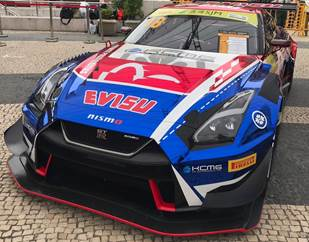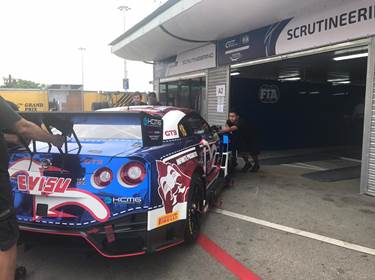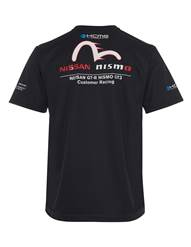年度最猛“哥斯拉”惊现澳门格兰披治大赛车

2018年11月19日 10:46 来源：车行天下 超过：14784次关注

EVISU携手KCMG赛车队出征澳门FIA GT世界杯2018年11月15日-日系街头潮牌EVISU与KGMG车队携手出战2018 FIA 澳门GT世界杯，该赛事为今年11月15日至18日举办的第65届澳门格林披治大赛车其中的一项重要赛事。KCMG赛车队联合EVISU共同打造有着 “哥斯拉”外号的 #18 Nissan GT-R Nismo GT3炫酷赛车征战此次赛事，并由屡次在亚洲卡雷拉杯夺冠的天才瑞士籍车手亚历山大Alexandre Imperator驾驭这辆“猛禽”出战。EVISU更是为了此次赛事推出了限量版的GT-R赛道系列产品，其中包括EVISU x KCMG Nismo GTR 限量T桖和mini #18 Nissan GT-R Nismo GT3赛车模型，该系列在EVISU官网及赛事现场贩售。EVISU x KCMG Nismo GTR 限量T桖限量版赛车模型

EVISU集团主席及行政总裁潘君临先生表示：”我们很高兴的能够与知名的KCMG赛车队合作，携手定制打造 Nissan”哥斯拉”GTR赛车。该跨界合作正好契合了EVISU品牌DNA不安于现状与永不放弃的精神，我们期待迎接本周赛事的胜利。”

KCMG创始人Paul Ip表示: ”KCMG非常高兴与日系潮流品牌EVISU合作，打造专属的#18 Nissan GT-R携手出战澳门FIA GT世界杯。我们团队一直热衷与对于产品拥有热爱与激情的品牌合作，我们很期待透过这次的密切合作，在此次澳门大赛中征服著名的东望洋赛道获得胜利”

知名瑞士籍赛车手Alexandre Imperatori : ”我非常高兴能够代表EVISU及KCMG出战这次的澳门FIA GT世界杯。与EVISU的合作充满了创新和活力，我非常兴奋。同时这里的赛事和赛道一直以来都非常吸引着我，我希望能够再次的赢得胜利。相信这次强大的合作系列将助力本场赛事，同时我也迫不及待释放出“哥斯拉”的咆哮!

EVISU作为街头潮流文化的先驱者，不安于现状、勇于冒险与永不放弃的精神正好与充满正能量的赛车领域不谋而合，持续不断推陈出新以大胆前卫的品牌理念为大家呈现更多面的EVISU。

EVISU官方微信公众号EVISU官方微博(@EVISU中国官方微博)EVISU故事

EVISU 于1991年在日本大坂创立，名字的灵感来自日本神话中的EBISU惠比寿(亦称惠比须、夷，日语：えびす)。品牌最初每天最多只能製作约十四条牛仔裤，并由人手在每条牛仔裤的后袋绘上海鸥图样，亦因为这 份对牛仔裤的热爱及对细节一丝不苟的态度，令EVISU很快便被有著极高要求的日本时尚界所喜爱，而且更掀起了一股席卷全世界的古著牛仔裤热潮。EVISU 二十年来的坚持及专注，为品牌赢尽口碑及好评，成为不少牛仔裤收藏家及潮流人士的最爱。享誉国际的同时，并没有减少品牌对品质的严格要求，而匠心独具的核心价值早已经根深蒂固，更成为牛仔裤史上的一个经典。

“BEFORE ANYONE DID ANYTHING EVISU DID EVERYTHING”

EVISU的标语灵感来自披头四成员约翰连侬(John Lennon)讚赏「摇滚之王」猫王的名句「Before anyone did anything, Elvis did everything」。猫王以其创意音乐及精彩表演，成为摇滚乐的经典人物。后来EVISU的创办人山根英彦先生借用此语录，改为EVISU的品牌标语。品牌2012年为庆祝EVISU成立20週年推出的成衣系列，亦使用这句标语。作为牛仔服饰的先驱，EVISU以独特砂洗工艺及精巧细节见称，并致力实践公司标语的精神，令EVISU成为牛仔服饰的不朽象徵。

EVISUKURO

EVISU在牛仔裤及服饰中常常藉由经典的图案及文化，向全球潮流人士展示日本的传统文化。同时间，亦希望透过大胆的设计元(报价 参数 图片 论坛)素展现其永不安于现状的态度。2016年，EVISU藉著品牌廿五週年之际，推出全新的EVISUKURO支线， 并以〝BEYOND BLACK〞及〝BEYOND DENIM〞作为口号打造出更富有冒险精神及青春玩味的服饰。BEYOND BLACK代表了EVISUKURO除主打黑白两色外，更会配合当季主题或流行的色调，提供多元化选择;至于BEYOND DENIM则代表了EVISUKURO的可变性时尚元素，将现今最流行的Hi-Street街头风与功能运动元素结合，推祟集休閒与时尚于一身的运动造型「ATHLEISURE LOOK」，为EVISU品牌历史开创全新的一页。

相关文章

0-500 字已有评论 0条 查看评论>>

﻿
• 快速找车
• 选择品牌
• 选择品牌
• A  奥迪
• A  阿斯顿·马丁
• A  阿尔法·罗密欧
• B  宝沃
• B  布加迪
• B  巴博斯
• B  保时捷
• B  宾利
• B  奔驰
• B  宝马
• B  本田
• B  别克
• B  标致
• B  比亚迪
• B  宝骏
• B  北汽制造
• B  北汽新能源
• B  北汽幻速
• B  北汽威旺
• B  北京汽车
• B  奔腾
• B  北汽绅宝
• C  长安
• C  长安商用
• C  长城
• C  昌河
• D  大众
• D  道奇
• D  DS
• D  东南
• D  东风风神
• D  东风风行
• D  东风小康
• D  东风风度
• D  东风
• F  福特
• F  丰田
• F  菲亚特
• F  法拉利
• F  福田
• F  福迪
• F  福汽启腾
• G  观致
• G  广汽传祺
• G  广汽吉奥
• G  GMC
• H  红旗
• H  汉腾汽车
• H  哈弗
• H  哈飞
• H  海格
• H  海马
• H  华颂
• H  黄海
• H  华泰
• H  恒天
• J  吉利汽车
• J  捷豹
• J  Jeep
• J  江淮
• J  江铃
• J  金杯
• J  九龙
• J  金旅
• K  凯翼
• K  凯迪拉克
• K  克莱斯勒
• K  科尼塞克
• K  卡威
• K  开瑞
• L  路虎
• L  林肯
• L  劳斯莱斯
• L  兰博基尼
• L  雷克萨斯
• L  铃木
• L  雷诺
• L  理念
• L  力帆
• L  莲花汽车
• L  猎豹
• L  路特斯
• L  陆风
• M  马自达
• M  MG
• M  MINI
• M  玛莎拉蒂
• M  摩根
• M  迈凯轮
• N  纳智捷
• O  欧宝
• O  讴歌
• O  欧朗
• Q  奇瑞
• Q  起亚
• Q  启辰
• R  日产
• R  荣威
• R  瑞麒
• S  三菱
• S  斯威汽车
• S  萨博
• S  smart
• S  斯柯达
• S  斯巴鲁
• S  思铭
• S  双龙
• S  上汽大通
• S  双环
• T  特斯拉
• T  腾势
• W  沃尔沃
• W  五菱汽车
• W  五十铃
• W  威兹曼
• W  威麟
• X  现代
• X  雪佛兰
• X  雪铁龙
• X  西雅特
• Y  一汽
• Y  英菲尼迪
• Y  英致
• Y  依维柯
• Y  野马汽车
• Y  永源
• Z  众泰
• Z  中华
• Z  中兴
• Z  知豆
• 选择车系
• 选择车系
• 车型对比
• 选择品牌
• 选择品牌
• A  奥迪
• A  阿斯顿·马丁
• A  阿尔法·罗密欧
• B  宝沃
• B  布加迪
• B  巴博斯
• B  保时捷
• B  宾利
• B  奔驰
• B  宝马
• B  本田
• B  别克
• B  标致
• B  比亚迪
• B  宝骏
• B  北汽制造
• B  北汽新能源
• B  北汽幻速
• B  北汽威旺
• B  北京汽车
• B  奔腾
• B  北汽绅宝
• C  长安
• C  长安商用
• C  长城
• C  昌河
• D  大众
• D  道奇
• D  DS
• D  东南
• D  东风风神
• D  东风风行
• D  东风小康
• D  东风风度
• D  东风
• F  福特
• F  丰田
• F  菲亚特
• F  法拉利
• F  福田
• F  福迪
• F  福汽启腾
• G  观致
• G  广汽传祺
• G  广汽吉奥
• G  GMC
• H  红旗
• H  汉腾汽车
• H  哈弗
• H  哈飞
• H  海格
• H  海马
• H  华颂
• H  黄海
• H  华泰
• H  恒天
• J  吉利汽车
• J  捷豹
• J  Jeep
• J  江淮
• J  江铃
• J  金杯
• J  九龙
• J  金旅
• K  凯翼
• K  凯迪拉克
• K  克莱斯勒
• K  科尼塞克
• K  卡威
• K  开瑞
• L  路虎
• L  林肯
• L  劳斯莱斯
• L  兰博基尼
• L  雷克萨斯
• L  铃木
• L  雷诺
• L  理念
• L  力帆
• L  莲花汽车
• L  猎豹
• L  路特斯
• L  陆风
• M  马自达
• M  MG
• M  MINI
• M  玛莎拉蒂
• M  摩根
• M  迈凯轮
• N  纳智捷
• O  欧宝
• O  讴歌
• O  欧朗
• Q  奇瑞
• Q  起亚
• Q  启辰
• R  日产
• R  荣威
• R  瑞麒
• S  三菱
• S  斯威汽车
• S  萨博
• S  smart
• S  斯柯达
• S  斯巴鲁
• S  思铭
• S  双龙
• S  上汽大通
• S  双环
• T  特斯拉
• T  腾势
• W  沃尔沃
• W  五菱汽车
• W  五十铃
• W  威兹曼
• W  威麟
• X  现代
• X  雪佛兰
• X  雪铁龙
• X  西雅特
• Y  一汽
• Y  英菲尼迪
• Y  英致
• Y  依维柯
• Y  野马汽车
• Y  永源
• Z  众泰
• Z  中华
• Z  中兴
• Z  知豆
• 选择车系
• 选择车系
• 选择车型
• 选择车型
• 意见反馈